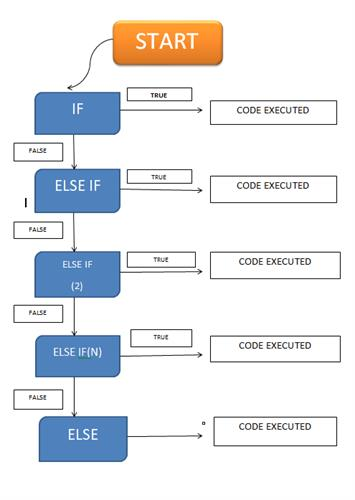Loading, please wait...# What are the Conditional Statements in C#

This article helps in understanding the various decision making statements in C# .

## CONDITIONAL STATEMENTS IN C#

CONDITIONAL STATEMENTS are the decision making statements in any programming language. In simple words, these statements enable the execution of a certain block of code to take place if a specified condition turns out to be true.

The various conditional statements are:

### If else statements:

These statements run on the basis of the evaluation of a specified condition in a Boolean form,i.e, either it should be true or false.

A condition is supplied to be checked in the if statement, and if it occurs to be true the block of code is executed, otherwise it is skipped.

Else if statement executes its block of code when a new condition specified turns out to be true.

Else statement is used when the condition is false in every case. So thereafter, the block of code associated with the else statement is executed.

Syntax:

``````if (test_condition1)
{
// block of code to be executed
}
else if(test_condition2)
{
// block of code to be executed
}
else if(test_conditionN)
{
// block of code to be executed
}
else
{
// block of code to be executed
}``````A program to illustrate the use of these statements is given below:

``````using System;

namespace ConsoleApp3
{
class Program
{
static void Main(string[] args)
{
Console.WriteLine("enter three numbers");

int n1 = Convert.ToInt32(num1);
int n2 = Convert.ToInt32(num2);
int n3 = Convert.ToInt32(num3);

Console.WriteLine("greater number is ");

if (n1>n2 && n1> n3)
{
Console.WriteLine(n1);
}
else if(n2>n1 && n2>n3)
{
Console.WriteLine(n2);
}
else
{
Console.WriteLine(n3);
}}}}
``````

### Switch statements:

C# includes another decision-making statement known as the switch statement. Switch statements are used where a list of values is compared and matched with a variable or an option selected by the user. These lists of values are termed as cases. Each case has its own block of code which is executed if the case value is matched with the user choice.

Also, each case has a break statement that is placed at the end which helps in termination of the execution of the code in that particular case.

Syntax:

``````switch(expression)
{
case
// block of code to be executed
break;

case
// block of code to be executed
break;

case
// block of code to be executed
break;

default:
// block of code to be executed
break;

}``````

A program to illustrate the use of the switch statement is given below:

``````using System;

namespace ConsoleApp2
{
class Program
{
static void Main(string[] args)
{
Console.WriteLine("ENTER FIRST NUMBER");

Console.WriteLine("ENTER SECOND NUMBER");

int n1 = Convert.ToInt32(num1);
int n2 = Convert.ToInt32(num2);

Console.WriteLine("enter operation to be performed");
Console.WriteLine("\n 1. Addition \n 2. Subtraction \n 3. Multiplication \n 4. Division");

int n3 = Convert.ToInt32(op);

switch(n3)
{
case 1:
{
Console.WriteLine(n1 + n2);
break;
}
case 2:
{
Console.WriteLine(n1 - n2);
break;
}
case 3:
{
Console.WriteLine(n1 * n2);
break;

}
case 4:
{
Console.WriteLine(n1 / n2);
break;
}
default:
Console.WriteLine("wrong choice entered");
break;
}
}
}
}
``````

### Ternary operator(?:)

The ternary operator is used in programs for decision-making purposes. It is of great significance because it reduces the size of the code due to its ability to be used in place of lengthy if-else statements.

The ternary operator takes three arguments in which the first argument is the comparison condition, the second argument is the outcome if the condition is true and the third argument is the outcome if the condition turns out to be false.

Syntax:

``condition? first statement : Second statement;``

A program to illustrate the use of the ternary operator  is given below:

``````using System;

namespace ConsoleApp4
{
class Program
{
static void Main(string[] args)
{
Console.WriteLine("enter number");